comparable 订阅
comparable是一个英语单词，形容词，作形容词时意为“类似的，可比较的；同等的，相当的”。 展开全文comparable是一个英语单词，形容词，作形容词时意为“类似的，可比较的；同等的，相当的”。

comparable

comparable单词发音

• 2020-01-05 22:30:20

接口介绍

功能:
所有可以排序的类都实现了 Comparable 接口
用于对集合内部元素进行排序
实现了 Comparable 的类 通过实现 comparaTo 方法 自定义排序规则
demo:
G:\project\demo\interface\Comparable
更多相关内容
• 本文，先介绍Comparable 和Comparator两个接口，以及它们的差异；接着，通过示例，对它们的使用方法进行说明
• 1.什么是Comparable接口 此接口强行对实现它的每个类的对象进行整体排序。此排序被称为该类的自然排序 ，类的 compareTo 方法被称为它的自然比较方法 。实现此接口的对象列表（和数组）可以通过 Collections.sort ...
• 下面小编就为大家带来一篇java中实现Comparable接口实现自定义排序的示例。小编觉得挺不错的，现在就分享给大家，也给大家做个参考。一起跟随小编过来看看吧java 自定义排序
• 主要介绍了java 实现Comparable接口排序,升序、降序、倒叙，具有很好的参考价值，希望对大家有所帮助。一起跟随小编过来看看吧
• NULL 博文链接：https://hellogava.iteye.com/blog/1570101
• 主要介绍了Java自然排序Comparable使用方法解析,文中通过示例代码介绍的非常详细，对大家的学习或者工作具有一定的参考学习价值,需要的朋友可以参考下
• 主要介绍了Java Comparable和Comparator对比详解,文中通过示例代码介绍的非常详细，对大家的学习或者工作具有一定的参考学习价值,需要的朋友可以参考下
• 主要介绍了java 中Comparable与Comparator详解与比较的相关资料,需要的朋友可以参考下
• 主要介绍了详解Java中Comparable和Comparator接口的区别的相关资料,希望通过本文大家能彻底掌握这部分内容，需要的朋友可以参考下
• Comparable和Comparator接口都可用作普通意义上对象间的比大小,但两个接口在实例化方面的用法不尽相同,接下来我们就来详细对比Java中的Comparable排序接口和Comparator比较器接口
• Java-中的-Comparator-和-Comparable.md
• java通过Comparable接口实现字符串比较大小排序的简单实例java
• 一种新的高效的基于请求的比较加密方案，陈鹏，叶俊，数据库中的加密数字比较是学术研究中的热点。尽管保序加密(OPE)可以达到这个目标,但是当一个范围内的所有数都用OPE来加密时,攻击者��
•   Comparable和Comparator都是两个接口，接口都可以用来实现集合中元素的比较、排序，Comparator位于包java.util下，而Comparable位于包java.lang下，Comparable接口将比较代码嵌入自身类中，而Comparator既可以...

### 一、概述

Comparable和Comparator都是两个接口，接口都可以用来实现集合中元素的比较、排序，Comparator位于包java.util下，而Comparable位于包java.lang下，Comparable接口将比较代码嵌入自身类中而Comparator既可以嵌入到自身类中，也可以在一个独立的类中实现比较。
Integer、String等这些基本类型的JAVA封装类都已经实现了Comparable接口，这些类对象本身就支持自比较，直接调用Collections.sort()就可以对集合中元素的排序，无需自己去实现Comparable接口。
而有些自定义类的List序列，当这个对象不支持自比较或者自比较函数不能满足你的要求时，你可以写一个比较器来完成两个对象之间大小的比较，也就是指定使用Comparator（临时规则排序，也称作专门规则排序），如果不指定Comparator，那么就用自然规则排序，这里的自然顺序就是实现Comparable接口设定的排序方式。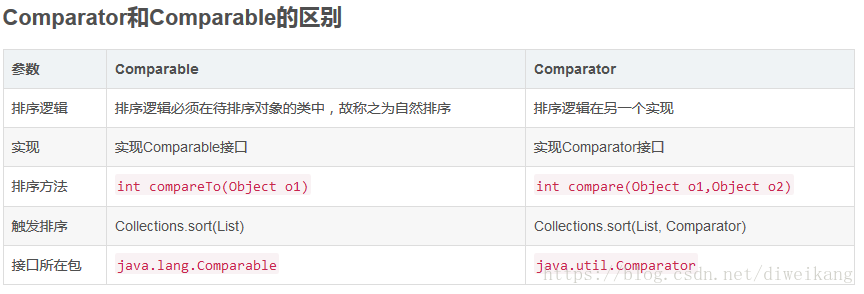### 二、Comparable

1、比较者大于被比较者（也就是compareTo方法里面的对象），那么返回正整数
2、比较者等于被比较者，那么返回0
3、比较者小于被比较者，那么返回负整数

### 三、Comparator

Comparator可以认为是是一个外比较器，个人认为有两种情况可以使用实现Comparator接口的方式：
1、一个对象不支持自己和自己比较（没有实现Comparable接口），但是又想对两个对象进行比较
2、一个对象实现了Comparable接口，但是开发者认为compareTo方法中的比较方式并不是自己想要的那种比较方式

Comparator接口里面有一个compare方法，方法有两个参数T o1和T o2，是泛型的表示方式，分别表示待比较的两个对象，方法返回值和Comparable接口一样是int，有三种情况：
1、o1大于o2，返回正整数
2、o1等于o2，返回0
3、o1小于o3，返回负整数

### 四、Comparator和Comparable的区别

一个类实现了Camparable接口则表明这个类的对象之间是可以相互比较的，这个类对象组成的集合就可以直接使用sort方法排序。
Comparator可以看成一种算法的实现，将算法和数据分离，Comparator也可以在下面两种环境下使用：
1、类的设计师没有考虑到比较问题而没有实现Comparable，可以通过Comparator来实现排序而不必改变对象本身
2、可以使用多种排序标准，比如升序、降序等

总结一下，两种比较器Comparable和Comparator，后者相比前者有如下优点：
1、如果实现类没有实现Comparable接口，又想对两个类进行比较（或者实现类实现了Comparable接口，但是对compareTo方法内的比较算法不满意），那么可以实现Comparator接口，自定义一个比较器，写比较算法
2、实现Comparable接口的方式比实现Comparator接口的耦合性要强一些，如果要修改比较算法，则需要修改Comparable接口的实现类，而实现Comparator的类是在外部进行比较的，不需要对实现类有任何修改。从这个角度说，实现Comparable接口的方式其实有些不太好，尤其在我们将实现类的.class文件打成一个.jar文件提供给开发者使用的时候。实际上实现Comparator 接口的方式后面会写到就是一种典型的策略模式。

### 五、实现方式

下面我们看一下具体实现方式：
1、Comparable
首先来看一下Comparable接口，我们想要对一个类自身进行排序，那么我们可以让这个类实现Comparable接口，重写其中的compareTo方法完成：

package cn.lxx.test;

public class User implements Comparable<User> {   //该类实现Comparable接口，参数为User
private String name;
private int age;
private boolean sex;

public User() {
}

public User(String name, int age, boolean sex) {
this.name = name;
this.age = age;
this.sex = sex;
}

@Override
public String toString() {
return "User{" +
"name='" + name + '\'' +
", age=" + age +
", sex=" + sex +
'}';
}

public String getName() {
return name;
}

public void setName(String name) {
this.name = name;
}

public int getAge() {
return age;
}

public void setAge(int age) {
this.age = age;
}

public boolean isSex() {
return sex;
}

public void setSex(boolean sex) {
this.sex = sex;
}

/**
* 重写compareTo方法，实现年龄升序,年龄相同则姓名升序
**/
@Override
public int compareTo(User user) {
if (this.age == user.age) return this.name.compareTo(user.name);
return this.age - user.age; //将this想像成一排不变的对象(已经按照要求排好序的)，而User就是当前要插入的对象，只有user属性小于this属性才插入从而升序，个人理解，希望有所帮助
}
}

class Test {
public static void main(String[] args) {
User user1 = new User("ake", 25, true);
User user2 = new User("reo", 24, false);
User user3 = new User("fg", 24, false);
List<User> list = new ArrayList<User>();
Collections.sort(list);
System.out.println("Comparable:" + list);
}
}


结果：
Comparable:[User{name='fg', age=24, sex=false}, User{name='reo', age=24, sex=false}, User{name='ake', age=25, sex=true}]
2、Comparator
在第二种实现方式Comparator接口中，总结了类自身实现Comparator接口重写compare方法的比较器、类外实现比较器和使用Lambda表达式简化比较器实现。



import java.util.ArrayList;
import java.util.Collections;
import java.util.Comparator;
import java.util.List;

public class User implements Comparator<User> {
private String name;
private int age;
private boolean sex;

public User() {
}

public User(String name, int age, boolean sex) {
this.name = name;
this.age = age;
this.sex = sex;
}

@Override
public String toString() {
return "User{" +
"name='" + name + '\'' +
", age=" + age +
", sex=" + sex +
'}';
}

public String getName() {
return name;
}

public void setName(String name) {
this.name = name;
}

public int getAge() {
return age;
}

public void setAge(int age) {
this.age = age;
}

public boolean isSex() {
return sex;
}

public void setSex(boolean sex) {
this.sex = sex;
}

@Override
public int compare(User o1, User o2) {
return o1.age-o2.age;
}
}
class Test{
public static void main(String[] args) {
User user1=new User("dingli",25,true);
User user2=new User("huxiaojuan",24,false);
User user3=new User("xxx",24,false);
List<User> list=new ArrayList<User>();
Collections.sort(list, new User()); //类实现了的Comparator能满足需求
System.out.println("类自身实现Comparator:"+list);
//现在我想要按照名字升序，显然类中实现的不能满足要求，于是可以在类外自己实现想要的比较器
Collections.sort(list, new Comparator<User>() {
@Override
public int compare(User o1, User o2) {
return o1.getName().compareTo(o2.getName()); //按照名字升序
}
});
System.out.println("匿名内部类方式："+list);
//由于Comparator接口是一个函数式接口，因此根据jdk1.8新特性，我们可以采用Lambda表达式简化代码
Collections.sort(list,(u1,u2)->{return u1.getName().compareTo(u2.getName());});
System.out.println("Lambda表达式方式："+list);
}
}


类自身实现Comparator:[User{name='reo', age=24, sex=false}, User{name='fg', age=24, sex=false}, User{name='ake', age=25, sex=true}]

匿名内部类方式：[User{name='ake', age=25, sex=true}, User{name='fg', age=24, sex=false}, User{name='reo', age=24, sex=false}]

Lambda表达式方式：[User{name='ake', age=25, sex=true}, User{name='fg', age=24, sex=false}, User{name='reo', age=24, sex=false}]

这里简单说一下什么是函数式接口，一般而言，函数式接口(Functional Interface)就是一个有且仅有一个抽象方法但是可以有多个非抽象方法的接口。@FunctionalInterface：标明一个接口是一个函数式接口。
接口概述：

接口中的方法会被隐式的指定为  public abstract
接口中的变量会被隐式的指定为  public static final


我们看一下上面Comparator使用到的Collections.sort的源码：

 @SuppressWarnings({"unchecked", "rawtypes"})
public static <T> void sort(List<T> list, Comparator<? super T> c) {
list.sort(c);
}


第二个参数是 Comparator<? super T> c点进去可以看到如下：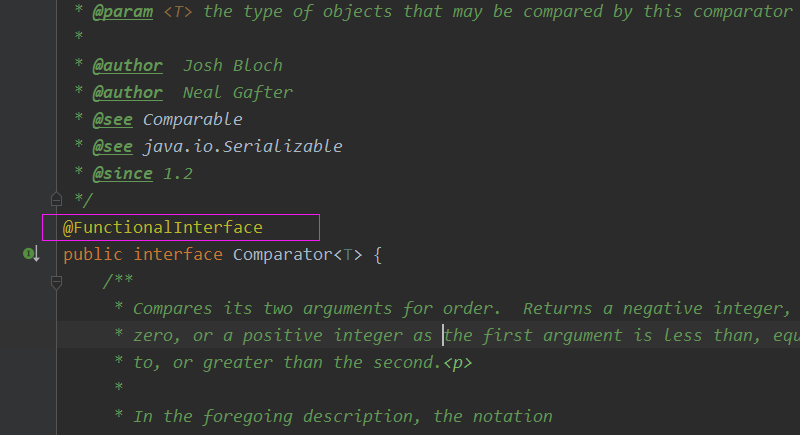发现他就是一个函数式接口，再看看compare方法要求(参数和返回值)，于是乎就可以使用Lambda表达式简化了。

展开全文java 算法 开发语言
• 给学生对象数组排序的两种方法——Comparable接口和Comparator接口大家好，我是小鱼儿

新的一天，大家一起加油!目录

引子

Comparable接口

# 引子

我们之前的文章的文章提到了Arrays是一个数组工具类，用Arrays.sort能够对各种类型的数组进行排序，那下面的数组能不能用Arrays.sort排序呢？

class Student {  // 自定义的学生类
String name;
int age;

public Student(String name, int age) {
this.name = name;
this.age = age;
}

@Override  // 对父类Object的父类方法进行重写，以便直接打印出当前对象的值
public String toString() {
// 这是IDEA的自动生成的toString重写，你也可以按照自己的喜好自由的选择打印的形式
return "Student{" +
"name='" + name + '\'' +
", age=" + age +
'}';
}
}
public class test4 {
public static void main(String[] args) {
Student[] students = new Student[] { // 我们定义了一个数组，数组中每个元素都是一个学生对象
new Student("zhangsan", 13),
new Student("lisi", 23),
new Student("able", 17),
};
Arrays.sort(students); // 用类Arrays.sort对students数组进行排序
System.out.println(Arrays.toString(students));
// 我们前几篇文章专门对Arrays以及toString方法的重写做了说明，这里不再赘述
}
}

但你运行的时候，你会发现程序报错了😮

对了，我记得sort只能对给定长度的数组排序，否则会发出空指针异常，但这里就是给定长度的数组啊！为啥还是错呢？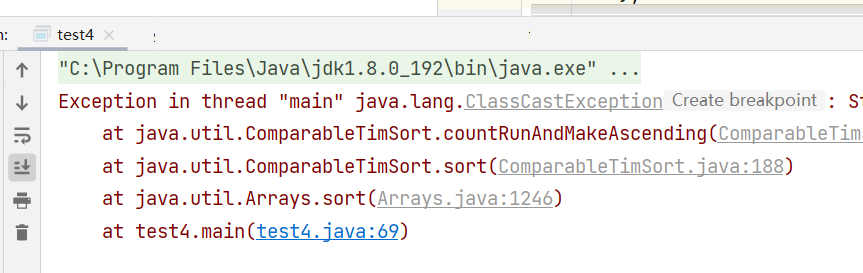程序抛出了异常😮，你说不对呀！我的Arrays不是对任意的数组都可以进行操作吗？之前我们就用Arrays.toString成功打印了数组元素都是对象的一个数组呀！

但是朋友，你告诉Arrays拿什么排了吗？是按姓名 还是按年龄呢？

🍑所以我们要告诉编译器那什么来排序，而这就要用到我们的Comparable接口

# Comparable接口

📝通过参考手册我们可以看到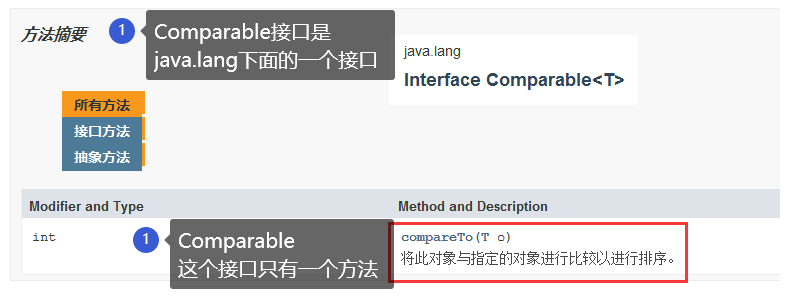我们的程序就可以写成这样

//Comparable接口在java.lang这个包下面，而java.lang这个包由java解释器自动引入，而不用import语句引入
class Student implements Comparable<Student>{  // 实现Comparable接口，注意Comparable后要加上要比较的对象的类型<Student>
String name;
int age;

public Student(String name, int age) {
this.name = name;
this.age = age;
}

@Override  // 对父类Object的父类方法进行重写，以便直接打印出当前对象的值
public String toString() {
return "[" + this.name + ":" + this.age + "]";
}

@Override // 实现Comparable接口中的中的compareTo方法
public int compareTo(Student o) {
if (this.age > o.age) return 1;  // this.代表对当前对象的引用，o.代表对参数对的引用
else if (this.age < o.age) return -1;
else return 0;
}
//如果当前对象应排在参数对象之前, 返回小于 0 的数字;
//比如上面我们如果this.age>o.age，那么返回1，就this.age在o.age的后面，即按年龄升序排列
//如果当前对象应排在参数对象之后, 返回大于 0 的数字;
//如果当前对象和参数对象不分先后, 返回 0;
}
public class test4 {
public static void main(String[] args) {
Student[] students = new Student[] { // 我们定义了一个数组，数组中每个元素都是一个学生对象
new Student("zhangsan", 13),
new Student("lisi", 23),
new Student("able", 17),
};
Arrays.sort(students); // 用类Arrays.sort对students数组进行排序
System.out.println(Arrays.toString(students));
// 我们前几篇文章专门对Arrays以及toString方法的重写做了说明，这里不再赘述
}
}

😁看一下输出结果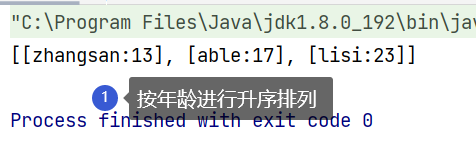📝咱们再回过头来看看为为啥必须要实现接口中的 compareTo 方法，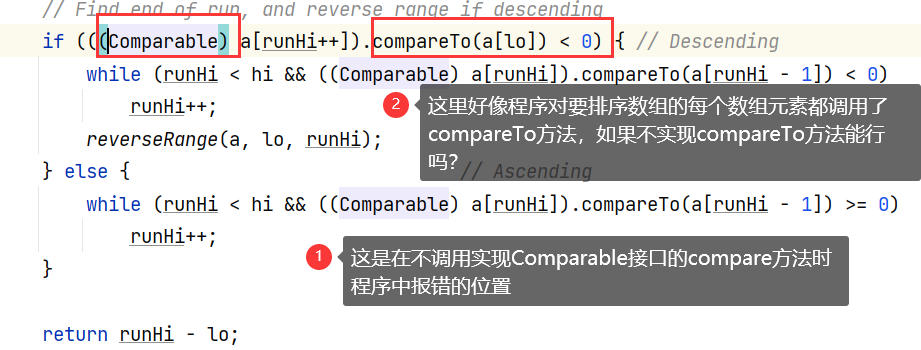上图中的这个（Comparable)a[runHi++]数组就是我们Arrays.sort传过去的学生对象数组🤔，下图中的this指的是谁🤔？就要看谁此时调用了compareTo方法😎

上图中compareTo(a[lo])点号前面的的学生对象a[runHi++]就是此时的this😮。下图中的o对象就是上图中的compareTo(a[lo]中的a[lo]学生对象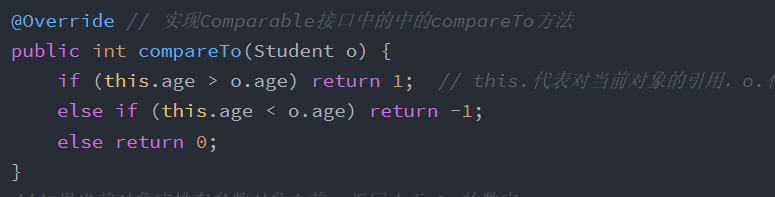不知道你理解了吗😂？如果没理解，我们不妨再举一个栗子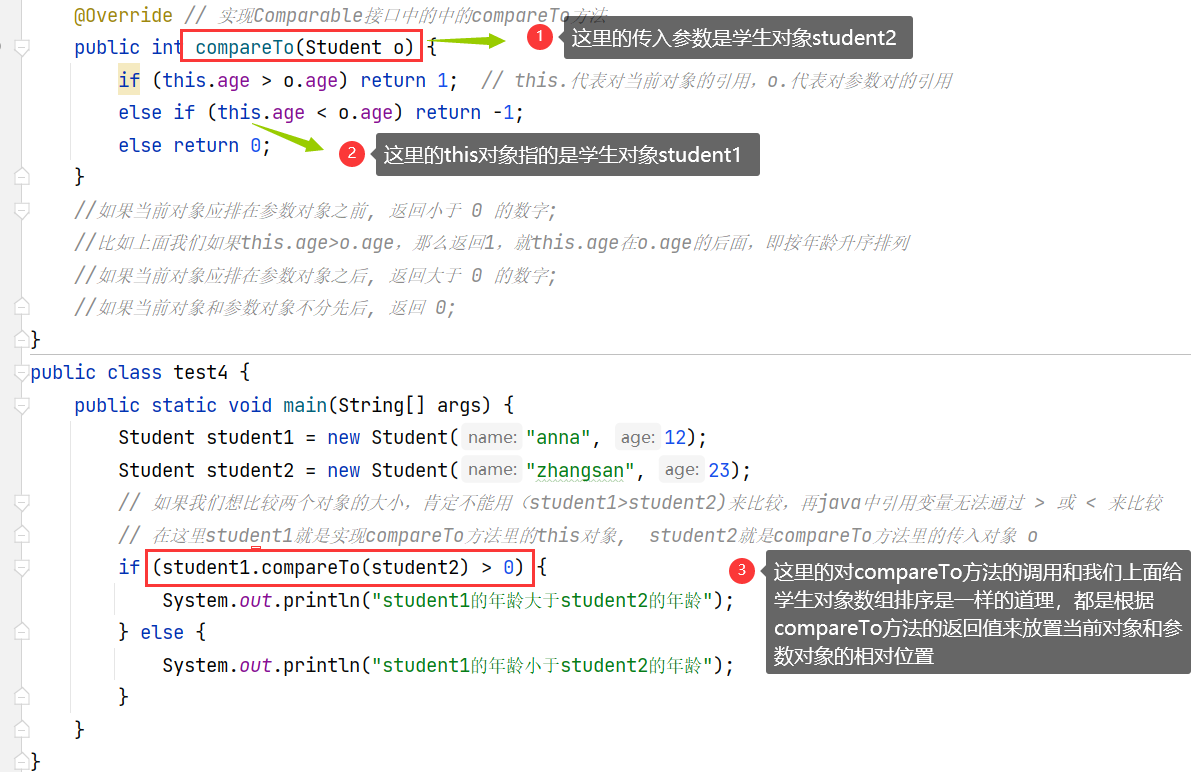🌰总结一下

📝我们知道在Arrays.sort(students); 中是传了一个学生对象数组，如上图所示在调用Arrays对对象数组排序的时候，其实就调用了我们的Comparable接口中的compareTo方法对数组的每个元素进行了排序😁

📝其实在Java中对于引用数据类型的比较或者排序，一般都要用到使用Comparable接口中的compareTo() 方法

🍑那如何按年龄排序呢？一样的道理（只是发生了一些小小的变化）

//Comparable接口在java.lang这个包下面，而java.lang这个包由java解释器自动引入，而不用import语句引入
class Student implements Comparable<Student>{  // 实现Comparable接口，注意Comparable后要加上要比较的对象的类型<Student>
String name;
int age;

public Student(String name, int age) {
this.name = name;
this.age = age;
}

@Override  // 对父类Object的父类方法进行重写，以便直接打印出当前对象的值
public String toString() {
return "[" + this.name + ":" + this.age + "]";
}

@Override // 实现Comparable接口中的中的compareTo方法
public int compareTo(Student o) {
// 为啥在compareTo里还要用compareTo，因为name是个String类型也是个引用类型，也需要用compareTo比较, 不过这里用到的compareTo方法是String类里重写的
if (this.name.compareTo(o.name) > 0) return 1;  // this.代表对当前对象的引用，o.代表对参数对的引用
else if (this.name.compareTo(o.name) < 0) return -1;
else return 0;
}
//如果当前对象应排在参数对象之前, 返回小于 0 的数字;
//如果当前对象应排在参数对象之后, 返回大于 0 的数字;
//如果当前对象和参数对象不分先后, 返回 0;
}
public class test4 {
public static void main(String[] args) {
Student[] students = new Student[] { // 我们定义了一个数组，数组中每个元素都是一个学生对象
new Student("zhangsan", 13),
new Student("lisi", 23),
new Student("able", 17),
};
Arrays.sort(students); // 用类Arrays.sort对students数组进行排序
System.out.println(Arrays.toString(students));
// 我们前几篇文章专门对Arrays以及toString方法的重写做了说明，这里不再赘述
}
}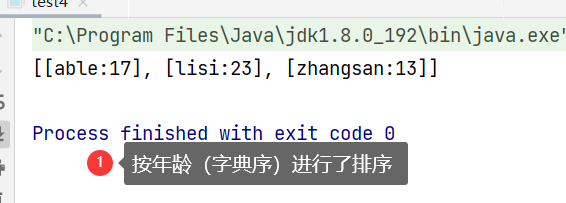bi

📝但这样会有一个问题，那就是一但写好了排序的方法，那就一下子就写死了😮。你在compareTo方法里实现的是按年龄比较，那你接下来就只能按年龄来比较🤔。如果想按姓名来比较，那只能够在重写compareTo方法。

🍑那有什么办法能够解决这个问题呢？

有！那就是我们接下来要讲的比较器：Comparator接口

# Comparator接口

🌰老规矩我们先来了解一下Comparator这个接口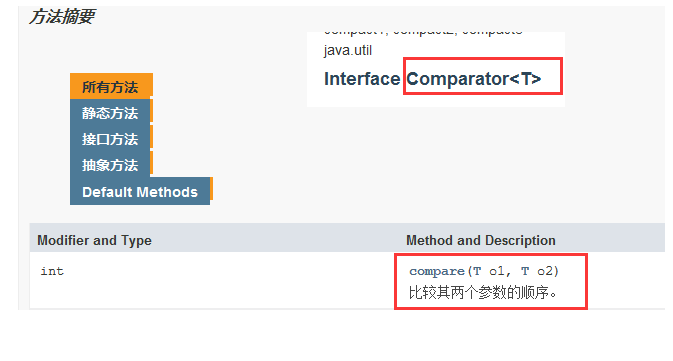所以就像Comparable 接口一样，我们只要实现了Comparator接口，并重写Comparator里的compare方法就可以实现对学生对象数组的排序

🌰比如我们上面的年龄比较就可以写成这样

class Student {
String name;
int age;

public Student(String name, int age) {
this.name = name;
this.age = age;
}

@Override  // 对父类Object的父类方法进行重写，以便直接打印出当前对象的值
public String toString() {
return "[" + this.name + ":" + this.age + "]";
}
}
class AgeComparator implements Comparator<Student> { // 年龄比较器
@Override  // 实现Comparator接口中的compare方法
public int compare(Student o1, Student o2) {
return o1.age - o2.age;
// 反正返回的也是数字，当o1.age>o2.age时返回大于零的数，即o1对象排在o2对象的后面，升序排列，我们之前用Comparable接口时也可以这样简写
}
//如果当前对象应排在参数对象之前, 返回小于 0 的数字;
//如果当前对象应排在参数对象之后, 返回大于 0 的数字;
//如果当前对象和参数对象不分先后, 返回 0;
}
public class test4 {
public static void main(String[] args) {
Student[] students = new Student[]{ // 我们定义了一个数组，数组中每个元素都是一个学生对象
new Student("zhangsan", 13),
new Student("lisi", 23),
new Student("able", 17),

};
AgeComparator ageComparator = new AgeComparator(); // 对年龄比较器进行实例化
Arrays.sort(students, ageComparator);
// 用类Arrays.sort对students数组进行排序，这里传了两个参数（学生对象和所对应的年龄比较器）
System.out.println(Arrays.toString(students));
// 我们前几篇文章专门对Arrays以及toString方法的重写做了说明，这里不再赘述
}
}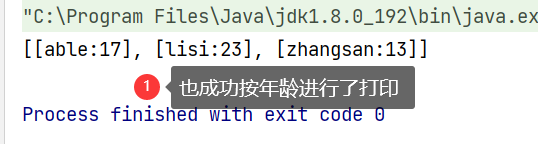📝Arrays.sort此时是一个传两个参数的方法：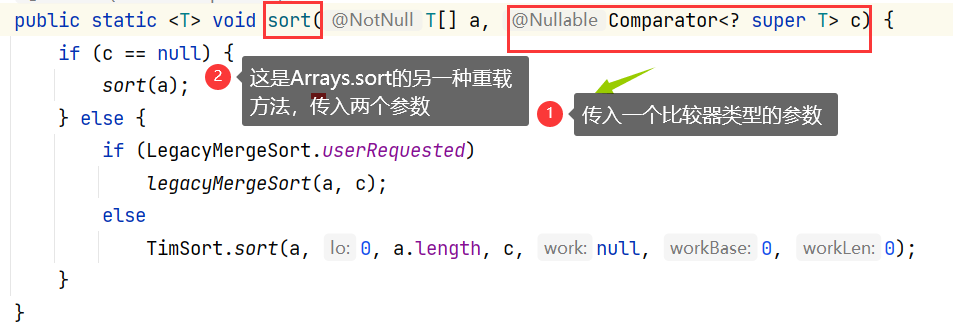🌰那姓名比较比较自然就出来了

class Student {
String name;
int age;

public Student(String name, int age) {
this.name = name;
this.age = age;
}

@Override  // 对父类Object的父类方法进行重写，以便直接打印出当前对象的值
public String toString() {
return "[" + this.name + ":" + this.age + "]";
}
}
class NameComparator implements Comparator<Student> { // 姓名比较器
@Override  // 实现Comparator接口中的compare方法
public int compare(Student o1, Student o2) {
return o1.name.compareTo(o2.name);
// 因为name是String类型，也是一个引用类型，也要用到compareTo方法，此时的compareTo方法是String类里重写的方法
}
//如果当前对象应排在参数对象之前, 返回小于 0 的数字;
//如果当前对象应排在参数对象之后, 返回大于 0 的数字;
//如果当前对象和参数对象不分先后, 返回 0;
}
public class test4 {
public static void main(String[] args) {
Student[] students = new Student[]{ // 我们定义了一个数组，数组中每个元素都是一个学生对象
new Student("zhangsan", 13),
new Student("lisi", 23),
new Student("able", 17),

};
NameComparator nameComparator = new NameComparator();
Arrays.sort(students, nameComparator); // 用类Arrays.sort对students数组进行排序
System.out.println(Arrays.toString(students));
// 我们前几篇文章专门对Arrays以及toString方法的重写做了说明，这里不再赘述
}
}


总结一下就是：

📝Comparable接口和Comparator接口都是Java中用来比较和排序引用类型数据的接口，要实现比较，就需要实现他们所各种对应的compareTo方法或者compare方法。

🌰不同的是：Comparator使用起来更加灵活，所以我更倾向于使用比较器：Comparator

但注意，sort只能对给定长度的数组排序，否则会发出空指针异常

好了，今天我们就聊到这里，咱们下篇博客见🥰展开全文数组排序 Java基础
• 基于事件抽取的中日新闻可比语料库构建，杨健，徐金安，平行双语语料库提供了两种相应语言的丰富的匹配信息。通常情况下，获得高质量和大规模平行双语语料库的难度较大。在本文中，我们
• Comparable的用法1---马克-to-win java视频的详细描述
• } /** * 数组元素元素i和j交换位置 */ private static void exch(Comparable[] a,int i,int j){ Comparable temp; temp=a[i]; a[i]=a[j]; a[j]=temp; } } 2.选择排序 public class Selection { /** * 对数组中的...

目录

1.冒泡排序

2.选择排序

3.插入排序

4.希尔排序

5.归并排序

6.快速排序

# 1.冒泡排序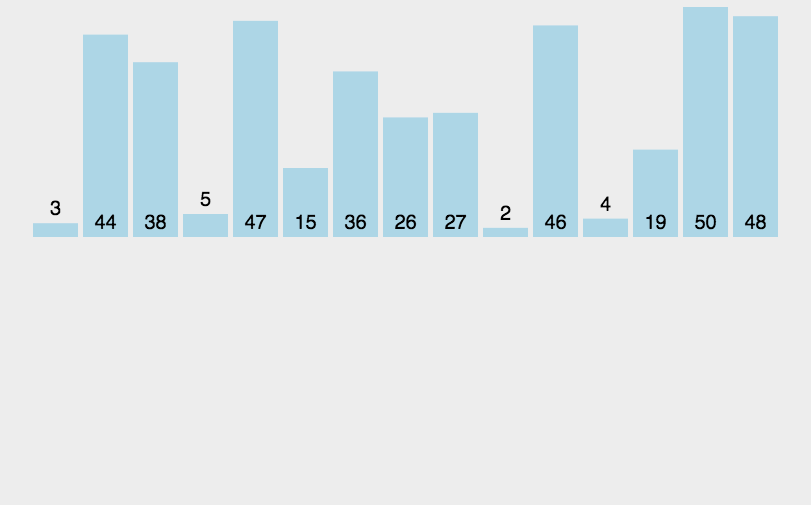//冒泡排序
public class Bubble {

/**
* 对数组a中的元素进行冒泡排序
*/
public static void sort(Comparable[] a){
for(int i=a.length-1;i>0;i--){
for(int j=0;j<i;j++){
//比较索引j与索引j+1处得值
if(greater(a[j],a[j+1])){
exch(a,j,j+1);
}
}

}
}

/**
* 比较v元素是否大于w元素
*/
private static boolean greater(Comparable v,Comparable w){
return v.compareTo(w)>0;
}

/**
* 数组元素元素i和j交换位置
*/
private static void exch(Comparable[] a,int i,int j){
Comparable temp;
temp=a[i];
a[i]=a[j];
a[j]=temp;
}

}

# 2.选择排序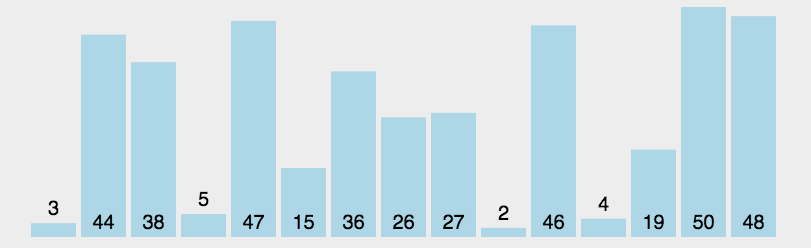public class Selection {

/**
* 对数组中的元素进行选择排序
*/
public static void sort(Comparable[] a){
for(int i=0;i<a.length-1;i++){
//定义一个变量，记录最小元素所在的索引，默认为参与选择的第一个元素所在的位置
int minIndex=i;
for(int j=i+1;j<a.length;j++){
//比较索引minIndex处的值与j索引的值
if(greater(a[minIndex],a[j])){
minIndex=j;
}
}
//交换最小元素所在索引minIndex处的值和索引i处的值
exch(a,i,minIndex);
}
}

/**
* 比较v元素是否大于w元素
*/
public static boolean greater(Comparable v,Comparable w){
return v.compareTo(w)>0;
}

/**
* 数组元素i和j交换位置
*/
public static void exch(Comparable[] a,int i,int j){
Comparable temp;
temp=a[i];
a[i]=a[j];
a[j]=temp;

}
}


# 3.插入排序public class Insertion {
/**
* 对数组a中元素进行插入排序
*/
public static void sort(Comparable a[]){
for(int i=1;i<a.length;i++){
for(int j=i;j>0;j--){
if(greater(a[j-1],a[j])){
exch(a,j-1,j);
}else{
break;
}
}
}
}

/**
* 比较v元素是否大于w元素
*/
public static boolean greater(Comparable v,Comparable w){
return v.compareTo(w)>0;
}

/**
* 数组元素i和j进行交换
*/
public static void exch(Comparable[] a,int i,int j){
Comparable temp;
temp=a[i];
a[i]=a[j];
a[j]=temp;
}
}


# 4.希尔排序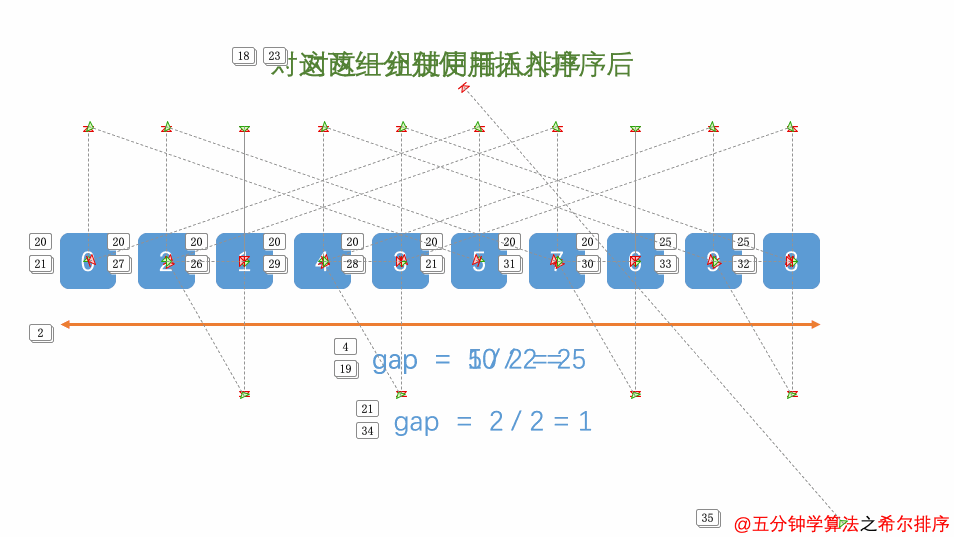public class Shell {
/**
* 对数组a进行希尔排序
*/
public static void sort(Comparable[] a){
//1.根据数组a的长度，确定增长量h的初始值
int h=1;
while(h<a.length/2){
h=2*h+1;
}
//2.希尔排序
while(h>=1){
//排序
//2.1.找到待插入的元素
for(int i=h;i<a.length;i++){
//2.2.把待插入的元素插入有序数列中
for(int j=i;j>=h;j-=h){
//待插入的元素是a[j],比较a[j]和a[j-h]
if(greater(a[j-h],a[j])){
exch(a,j-h,j);
}
else{
//待插入元素已经找到合适位置，退出循环
break;
}
}
}
//减小h的值
h=h/2;
}
}

/**
* 比较v和w大小
*/
public static boolean greater(Comparable v,Comparable w){
return v.compareTo(w)>0;
}

/**
* 数组元素i和j交换
*/
public static void exch(Comparable[] a,int i,int j){
Comparable temp;
temp=a[i];
a[i]=a[j];
a[j]=temp;
}
}


# 5.归并排序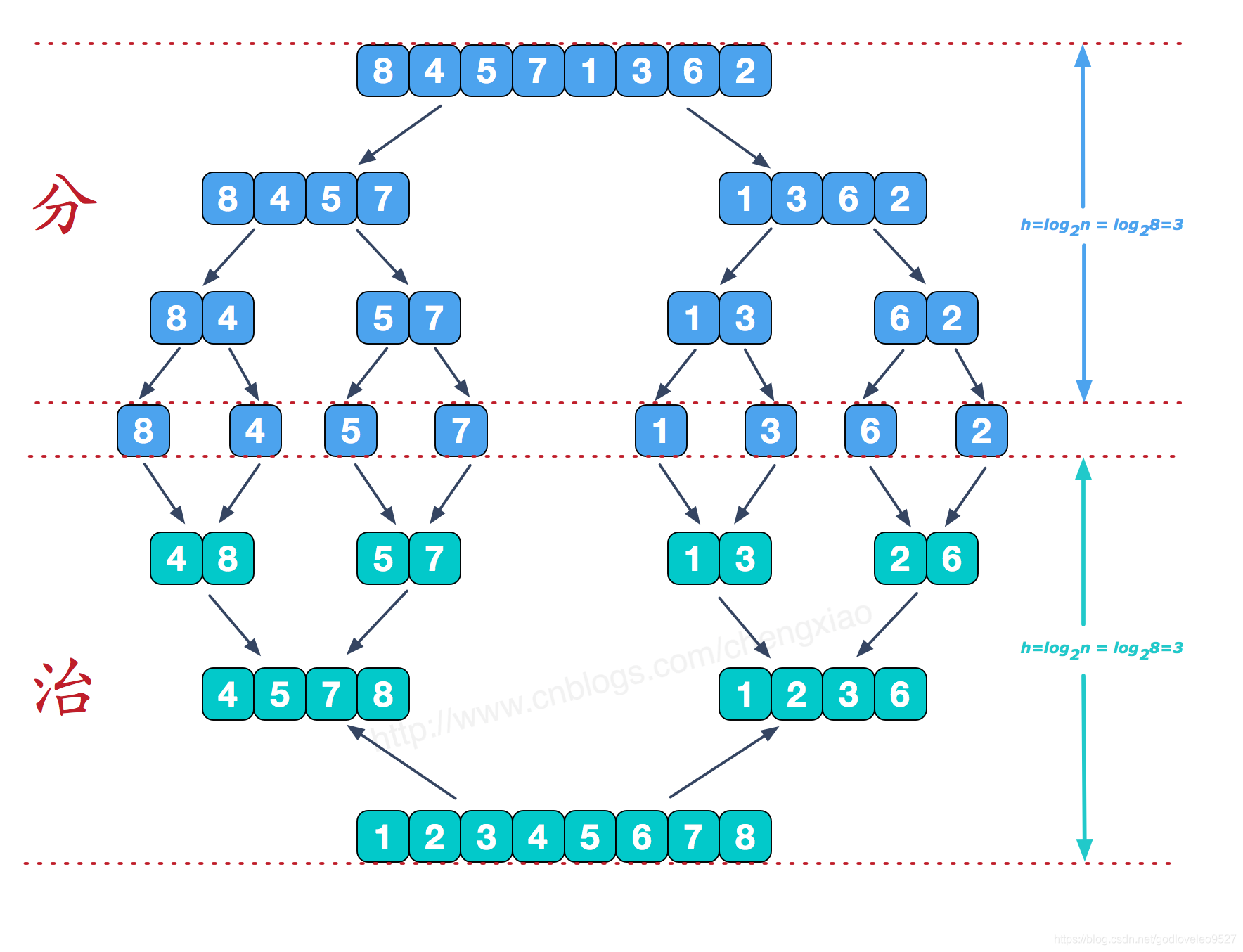public class Merge {

//归并所需要的辅助数组
private static Comparable[] assist;

/**
* 比较v元素是否小于w元素
*/
private static boolean less(Comparable v,Comparable w){
return v.compareTo(w)<0;
}

/**
* 数组元素i和j交换位置
*/
private static void exch(Comparable[] a,int i,int j){
Comparable temp;
temp=a[i];
a[i]=a[j];
a[j]=temp;
}

/**
* 对数组a中的元素进行排序
*/
public static void sort(Comparable[] a){
//1.初始化辅助数组 assist
assist=new Comparable[a.length];
//2.定义一个lo和hi变量，分别记录数组中最小的索引和最大的索引
int lo=0;
int hi=a.length-1;
//3.调用sort重载方法完成数组a中，从索引lo到hi的元素的排序
sort(a,lo,hi);
}

/**
* 对数组中lo到hi的元素进行排序
*/
public static void sort(Comparable[] a,int lo,int hi){
//做安全性校验
if(hi<=lo){
return;
}
//对lo到hi之间数据进行分组
int mid=lo+(hi-lo)/2;   //5 9    mid=7
//分别对每一组数据进行排序
sort(a,lo,mid);
sort(a,mid+1,hi);
//再把两个组的数据进行归并
merge(a,lo,mid,hi);
}

/**
* 对数组中，从lo到mid为一组，从mid+1到hi为一组，对这两组数据进行归并
*/
public static void merge(Comparable[] a,int lo,int mid,int hi){
//定义三个指针
int i=lo;
int p1=lo;
int p2=mid+1;
//遍历，移动p1指针和p2指针，比较对应索引处的值，找出小的那个，放到辅助数组的对应索引处
while(p1<=mid && p2<=hi){
//比较对应索引处的值
if(less(a[p1],a[p2])){
assist[i++]=a[p1++];
}else{
assist[i++]=a[p2++];
}
}
//遍历，如果p1指针没有走完，那么顺序移动p1指针，把对应的元素放到辅助数组对应索引处
while (p1<=mid){
assist[i++]=a[p1++];
}
//遍历，如果p2指针没有走完，那么顺序移动p2指针，把对应的元素放到辅助数组对应索引处
while(p2<=hi){
assist[i++]=a[p2++];
}
//把辅助数组中的元素拷贝到原数组中
for (int index=lo;index<=hi;index++){
a[index]=assist[index];
}
}

}


# 6.快速排序public class Quick {
/**
* 比较v是否小于w
*/
public static boolean less(Comparable v,Comparable w){
return v.compareTo(w)<0;
}

/**
* 交换数组元素i和j的位置
*/
public static void exch(Comparable[] a,int i,int j){
Comparable temp=a[i];
a[i]=a[j];
a[j]=temp;
}

/**
* 对数组内的元素进行排序
*/
public static void sort(Comparable[] a){
int lo=0;
int hi=a.length-1;
sort(a,lo,hi);
}

/**
* 对数组中元素从索引lo到hi之间的元素进行排序
*/
public static void sort(Comparable[] a,int lo,int hi){
//安全性校验
if (hi<=lo){
return;
}
//需要对数组中lo索引到hi索引处的元素进行分组（左子组，右子组）
int partition=partition(a,lo,hi); //返回的是分组的分界值所在的索引，分界值变换后的索引
//左子组有序
sort(a,lo,partition-1);
//右子组有序
sort(a,partition+1,hi);
}

/**
* 对数组a中，从索引lo到hi之间的元素进行分组，并返回分组界限对应的索引
*/
public static int partition(Comparable[] a,int lo,int hi){
//确定分界值
Comparable key=a[lo];
//定义两个指针，分别指向待切分元素最小索引处和最大索引处的下一个位置
int left=lo;
int right=hi+1;
//切分
while(true){
//先从右往左扫描，移动right指针，找到比分界值小的元素，停止
while(less(key,a[--right])){
if(right==lo){
break;
}
}
//从左往右扫描，移动left指针，找到比分界值大的元素，停止
while(less(a[++left],key)){
if (left==hi){
break;
}
}
//判断left>=right，如果是，则证明元素扫描完毕，结束循环，如果不是，则交换元素即可
if (left>=right){
break;
}else{
exch(a,left,right);
}
}
//交换分界值
exch(a,lo,right);
return right;
}
}


展开全文java 排序算法
• 1. 需要被排序的对象实现Comparable 接口，所以可以认为比较的属性是对象本身已经有了的，所以我们将由Comparable 实现的排序叫做自然排序 2. 当Comparable 实现的排序不能满足需求的时候，你可以使用Comparator ...编程语言 java
• Comparable & Comparator接口都可以用来实现集合中元素的比较、排序，Comparator位于包java.util下， Comparable位于包java.lang下 Comparable接口将比较代码嵌入自身类中 Comparator在一个独立的类中实现比较。...
• 第十二条： 考虑实现Comparable接口(注意本文不适合学习，纯属个人笔记)1. Comparable接口介绍一个类，如果实现了Comparable接口，我们可以很容易的将它在数组或是集合中进行排序。so。。。Comparable这个接口是用来...
• Comparable 和 Comparator 接口的存在就是为了对象比较，我们可以在接口中定义对象比较规则，还可以利用 Collections.sort 和 Arrays.sort 方法对对象数组和集合进行排序。 Comparable接口 Comparator接口 ...Java 自然排序
• $npm install comparable-video-viewer // with yarn$ yarn add comparable-video-viewer 运行示例 // build $yarn && yarn build // run example$ yarn && yarn build && yarn start-demo 源视频 源视频应并排...HTML
• 文章目录运行环境一、前言二、概要三、Comparable3.1 通过实现Comparable接口完成学生类的排序3.1.1 按成绩排序3.1.2 按身高排序3.1.3 总结Comparable四、Comparator 比较器4.1 通过Comparator比较器实现排序4.2 ...java
• ## java中Comparable接口

千次阅读 2021-03-06 15:47:01
public class CompareDemo implements Comparable{ private String name; private int age; public CompareDemo(){ } public CompareDemo(String name,int age){ this.name=name; this.age=age; } public void set...
• 一、java.lang.Comparable接口简介 1.1、Comparable接口应用背景 public final class Integer extends Number implements Comparable {...} 今天在开发中无意看到Integer包装类内部实现了Comparable接口，因此探查...
• JAVA中的Comparable 和 Comparatorjava 开发语言...